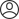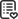SavedLevel

All Level

## Description

The comments pour in like start with Python or R programming courses or to take some data science courses on YouTube, Udemy, Coursera etc. We are not saying that these answers are absolutely wrong or that they are trying to promote something over the other but while answering the question they tend to skip the most important factor. That is paying attention to the student’s background. In general, these responses position Data Science as a programming or algorithmic field.

Not only this, but we have also observed questions based on visualisation and modelling results produced with Python or R. Many of which tend to show missing basic understanding to statistics.

It is very important to realise that all these specialization domains like Data Science, Data Analytics, Data Engineers etc, have the word Data in it. If we go by the definition of the Statistics it states,

Statistics is the Heart of all these New Specialization Fields.

Our suggestions for a new learner, is to start by learning statistics and building a sound knowledge of it. You can take such courses with any elearning schools or from qualified instructors with a background in Statistics.

Some of the topics you will learn are:

• Statistics, data and statistical thinking
• Types of data
• Basic notions of samples and populations
• Methods for describing quantitative data and qualitative data
• Counting techniques (Permutations and Combinations)
• Probability
• Discrete Random variables
• Continuous random variables (Normal distribution)
• Sampling Distributions
• Inferences based on a single sample (Confidence intervals and tests of hypotheses)
• Inferences based on two samples
• ANOVA (Analysis of Variance)
• Correlations and Simple Linear Regression
• Multiple regression
• Basic categorical data analysis
• This basic statistics course will provide you the right foundation to start learning other Machine Learning topics.

It is also recommended to have some basic math skills such as algebra, calculus and linear algebra.

After taking the statistics course, the next course you can choose are SQL and Spark SQL. You will require strong SQL skills in order to extract and analyze large datasets. Python and R are needed programming languages. You can start with Python first. Overtime, there could be some cases where you need to learn R because it’s the most complete statistical programming language.